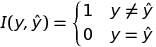# Loss Function

## What is a loss function?

Loss functions are used to determine the error (aka “the loss”) between the output of our algorithms and the given target value.  In layman’s terms, the loss function expresses how far off the mark our computed output is.

### Common Loss Functions

There are multiple ways to determine loss.  Two of the most popular loss functions in machine learning are the 0-1 loss function and the quadratic loss function.  The 0-1 loss function is an indicator function that returns 1 when the target and output are not equal and zero otherwise:

0-1 Loss:The quadratic loss is a commonly used symmetric loss function.  The quadratic losses’ symmetry comes from its output being identical with relation to targets that differ by some value x in any direction (i.e. if the output overshoots by 1, that is the same as undershooting by 1).  The quadratic loss is of the following form: